알림

[Python Studies] 2. Arithmetic Operators and Assignment OperatorWe call them computers because they 'compute', so thus Python. Open your IDLE and type in some basic arithmetic operations.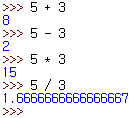Seems like it knows how to do some work. Actually, aside from these four operations, Python can do three more.The first equation returns the remainder, the second one returns the quotient and the last one returns the power.

These kinds of expressions consist of two parts. The value, and the operator. If the expression misses any of these or the value is less or the same as the operator, an error will occur.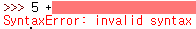Just like mathematics, we have to know which operators are executed before which.

1. **
2. * / % //
3. + -

Let's see some examples.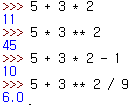However, even though you know the precedence, it's much better if you use parentheses to indicate precedence. It's not just because it can prevent ambiguousness but it can be easy to understand for many more people who read it which includes you.

Sometimes when we do operations, we use 'variables'. In Python, we can do that. See the following example.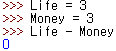Philosophical, isn't it?

Now, the equal sign that assigns the value is called 'assignment operator'. You can also use expressions when assigning.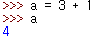We also can do special tricks.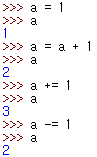Can you get the trick inside? First, we can use the same variable to change the value of it. Second, we can use such trick more easily by adding an arithmetic operator right before the assignment operator.

We can also change the value of the variable by assigning another variable.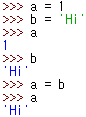You can see that a was originally a number, and b is a string. But after assigning b to a, a's value has been changed to b's value.